# Research on Residual Stress Quantitative Reduction in Laminar Cooling on Hot Strip Mill

Research on Residual Stress Quantitative Reduction in Laminar Cooling on Hot Strip Mill

Wenquan SunJian Shao Anrui He Haishan Zhao Jie Zhou

National Engineering Research Center of Advanced Rolling Technology, University of Science and Technology, Beijing, China

No.1 Steel Making and Rolling Plant, Maanshan Iron & Steel Company Ltd., China

Corresponding Author Email:
wqsun18@163.com
Page:
19-24
|
DOI:
http://dx.doi.org/10.18280/ijht.330403
|
Accepted:
|
Published:
31 December 2015
| Citation

OPEN ACCESS

Abstract:

Laminar cooling technology is widely used in hot rolling process, especially, the ultra-fast cooling and dense cooling technologies play an important role in high strength steel strip production. Research on temperature and stress coupled model in the process of hot rolling laminar cooling is of positive significance for solving strip uneven transverse cooling, uneven stress and buckling. By using finite element method, temperature and stress coupled model of laminar cooling on hot strip mill is established, and the coupling calculation of temperature, phase transformation and stress is realized. Temperature test, material microstructure test and stress test are used for model validation. The result shows that strip transverse initial temperature difference and edge masking are significant to reduce residual stress. In this paper, an example is given to improve the strip residual stress by improving the initial temperature difference distribution, and the correctness of the proposed method and conclusion is verified.

Keywords:

Hot rolling, Laminar cooling, Multi-field coupling, FEM, Residual stress.

1. Introduction

In recent years, laminar cooling has been widely used in hot rolling process, especially, the ultra-fast cooling and dense cooling technologies play an important role in high strength steel strip production  . Using a new generation laminar cooling technology can enable strip rapid cooling to phase transformation zone, stop the growth of austenite grain, reduce the grain size and enhance the intensity of strip after finish rolling, also it can effectively reduce the alloy in strip and realize the cost reduction. According to the high cooling efficiency of new generation cooling, the uneven transverse cooling of strip, uneven stress and buckling are more serious than normal cooling technology .

There are many studies on temperature and phase transformation in laminar cooling. Wang, and Chen used the finite element method for temperature, phase transformation and stress calculation, but the researches are lack of sufficient experimental support  . Yi used experimental methods for residual stress calculation . Yoshida analyzed the residual stress of strip after laminar cooling, but the influence by different factors on residual stress is not concerned . Park studied phase transformation kinetics in strip laminar cooling and got the transformation kinetics equations, but the influence made by phase transformation on shape was not referred . Han and Lee also studied the residual stress during laminar cooling and deduced the constitutive equation, analyzed possibly shape problems in laminar cooling, but no effective optimization method was proposed .

To today, there are some residual stress reduction methods, such as two-stage cooling, sparse cooling and so on. Although these methods can reduce the residual stress in a certain extent, but it will lead to low strip strength because of coarse grain and also seriously affect the performance of high strength steel. In processing of hot strip, experimental study also cannot be unlimited according to quality and quantity. In this paper, the finite element method (FEM) is used to simulate the laminar cooling, which can achieve various results by modifying the boundary conditions freely. The finite element method studies influence factors of residual stress reduction in high strength strip, which can simulate cooling condition and replace the online testing, so that the cost and time can be greatly reduced. The simulation result can be used to guide high strength steel production.

2. FEM Model of Laminar Cooling

FEM model of laminar cooling consists of two parts: part one is a general finite element module using software ABAQUS, which includes computer aided design (CAE), material properties, meshing, boundary conditions and so on. The other part is a subroutine programmed by FORTRAN, which includes  phase transformation, constitutive equation et al. By using ABAQUS solver including subroutine, coupling calculation of temperature, phase transformation and stress is achieved.

2.1 CAE model of strip

Taking a 700Mpa high strength strip produced by a certain iron and steel company for example, the CAE of strip finite element model is shown in Fig.1. In CAE model, strip length is 6m, strip width is 1.2m and strip thickness is 0.003m. Mesh type is temperature and displacement coupled hexahedral elements coupled, corresponding to C3D8T unit in ABAQUS. Because the temperature field and stress field in the strip edge are more complex, so the mesh density of the 0.2m in the edge mesh is 5 times than other strip area.

## 1.png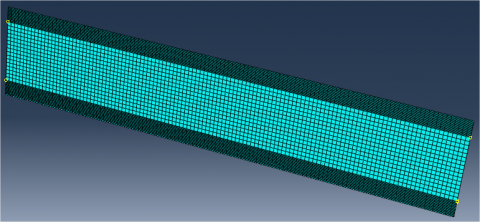Figure 1. CAE of strip finite element model

2.1 Material properties

In finite element simulation, the material physical parameters have great influence on the calculation process and results. Therefore, it is necessary to get the accurate parameters and add them to the finite element simulation model. Based on the components C (0.063%), Mn (1.843%), Si (0.019%), P (0.001%), s (0.001%), Ni (0.084), (0.062%) of Nb, Ti (0.094%), Cr (0.021%), Mo (0.172%), V (0.003%), temperature conduction rate, line expansion coefficient and specific heat of BS700 are achieved by experimental determination and reference. Because the analysis process is a temperature drop with a high temperature, the material parameters need to be considered in different temperature by interpolation calculation in ABAQUS.

2.2 Initial temperature and boundary conditions

Due to the strip thickness is very thin, the temperature and stress distribution in strip length and thickness direction are neglected. In heat transfer analysis of laminar cooling, initial temperature mainly refers to transverse uneven temperature distribution after finishing mill and it can be obtained by using thermal imager as shown in Fig.2. Temperature distribution of strip is fitted with six order curve and each position temperature along the strip width is achieved, in middle of strip, the temperature is uniform and big temperature drop is in the strip edge area.

## 2.jpg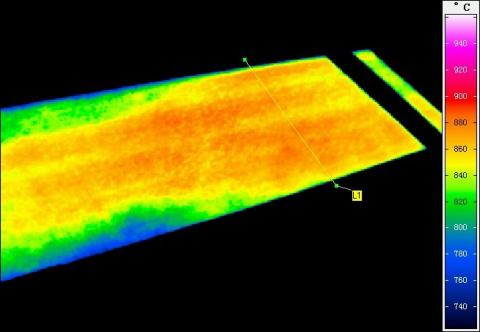Figure 2. Temperature distribution after finishing mill by using thermal imager

The boundary conditions of hot strip laminar cooling are complex, which mainly include convection heat transfer and strip heat radiation. Convection heat transfer occurred between strip and water, strip and air. In laminar cooling process, jet impingement and steady film boiling region are in strip surface, the convection heat transfer coefficient of the strip surface is mainly influenced by the equipment condition, cooling water quantity and the surface temperature of the strip.

2.3 Phase transformation model

Phase transformation model includes phase transformation kinetics, phase transformation heat calculation and phase incubation period calculation. Undercooling is power of phase transformation and phase transformation heat affect strip temperature, so phase transformation and strip temperature must be coupled solution.

Taking a 700Mpa high strength steel for example, austenite isothermal transformation kinetics can be expressed by Johnson-Mehl-Avrami-Kolmorgorov equation as follow:

$\frac{X}{X^{e}}=1-\exp \left(-b t^{n}\right)$      (1)

Where, X is phase transformation ratio, Xe is thermodynamic equilibrium fraction which is related to strip composition and temperature, t is the time, b is constant. The equation describes the austenite isothermal transformation process is obtained by the Scheil additive rule:

$\left.\begin{array}{rl}\frac{X_{i}}{X_{i}^{e}} & =1-\exp \left[-k_{i}\left(t^{\prime}+\Delta t\right)^{n}\right] \\ t^{\prime} & =\left[-\frac{1}{k_{i}} \ln \left(1-\frac{X_{i-1}}{X_{i}^{e}}\right)\right]^{1 / n}\end{array}\right\}$      (2)

Where Δt is time increment of i step, k and n are continuous non isothermal transformation parameters. Since ABAQUS is general finite element software without phase transformation calculation module, the ABAQUS is required to redevelop, in which the phase transformation model is written in sub program by using FORTRAN language.

2.4 Material constitutive relation

In hot rolling laminar cooling process, due to the different reasons, the strain is composed of several parts which can be expressed as follow:

$d \varepsilon_{i j}=d \varepsilon_{i j}^{\mathrm{e}}+d \varepsilon_{i j}^{\mathrm{th}}+d \varepsilon_{i j}^{\mathrm{p}}+d \varepsilon_{i j}^{\mathrm{tr}}+d \varepsilon_{i j}^{\mathrm{tb}}$      (3)

Where dεeij is the increment of elastic strain, dεthij is the increment of thermal strain, dεpij is the increment of plastic strain, dεtrij is the increment of strain coursed by phase transformation volume change, dεtbij is the increment of strain coursed by transformation induced plasticity.

$d \sigma_{i j}=[\mathrm{C}]_{i j} d \varepsilon_{i j}$    (4)

Where [C]ij is the stiffness matrix.

3. Model Validation by Expriment

3.1 Strip temperature field test in laminar cooling

Temperature calculation accuracy in laminar cooling is the basis of phase transformation and stress calculation. In order to obtain the real strip temperature during the whole cooling processing, in the laminar cooling there are four continuous temperatures measurement location by using thermal imager, which are behind last finishing stand, behind the first water cooling segment, front the second cooling segment and front coiling machine, where the strip transverse temperature can be achieved. As shown in Fig.3, temperature in strip transverse is compared by FEM model and measurement behind the first water cooling segment. It can be seen that the temperature calculation by finite element method is close to the actual measurement result, which is available for phase transformation and stress calculation.

## 3.pngFigure 3. Temperature compared in strip transverse behind the first water cooling segment

3.2 Phase transformation test

The CCT (Continuous Cooling Transformation) curves of the 700MPa high strength steel are obtained by Gleeble experiment, as shown in Figure 4. It can be seen from the diagram that the austenite is transformed into ferrite in high temperature section. In the case of low temperature and slow cooling rate, the austenite is transformed into bainite.

## 4.pngFigure 4. CCT curves of the 700MPa high strength steel

The phase transformation achieved by finite element model is shown in Fig.4, two nodes in middle and edge strip are chosen for analyzing the change proportion of austenite and ferrite in laminar cooling. It can be seen from the Fig.5 that phase transformation time in the edge of the strip is earlier than other zone due to the lower temperature and fast cooling rate. In laminar cooling, the phase transformation speed is always faster than the middle part. At the end of the transformation, the austenite in the middle part is transformed into ferrite completely and in the edge of strip, a large amount of ferrite is generated with a few other structures. Calculation data show that there is small amount of bainite in the edge of strip which is similar to CCT curve obtained experiment.

## 5.pngFigure 5. Phase transformation in middle and edge strip calculated by finite element model

In order to verify the phase transformation calculated by finite element model, different positions along the strip width are sampled and the structures are obtained by using scanning electron microscope (SEM) as shown in Fig.6 and Fig.7. The results of SEM show that there are ferrite and a small amount of carbide in middle strip, while in the strip edge, there are ferrite, carbides and bainite, which is relative to the lower temperature and fast cooling rate in the edge area and similar to the calculation result. The results of CCT curve and scanning electron microscope show that finite element model is accurate.

## 6.jpgFigure 6. Structures in middle strip by SEM

## 7.jpgFigure 7. Structures in edge strip by SEM

3.2 Residual stress measurement

The residual stress is measured by X-ray diffract meter as shown in Fig.8, which can avoids the influence of the mechanical stress caused by the small hole method in the process of drilling. X ray detection method is used to reflect the original state of the strip residual stress, and the detection results are relatively accurate.

## 8.png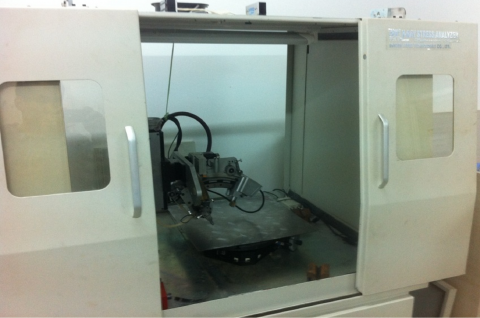Figure 8. X-ray diffract meter for residual stress measurement

Each measurement point can choose two or more measurement angle. The more measuring angle is, the more time consumes. It may take five minutes for each measurement, considering the cost and accuracy, four angles are used for residual stress measurement. According to the four results, residual stress is achieved. Due to the edge wave in the strip, residual stress in strip edge is more concerned. Measurement in strip edge is more densely, and in middle strip measurement is relatively sparse. Residual stress along the strip length direction calculated by FEM and measured by X-ray diffract meter are shown in Fig.9, which are more similar. It can be seen from the Fig.9 that strip central stress is positive and tensile stress, otherwise, in strip edge, the stress is negative, which reflects the edge wave in strip after cooling. The residual stress calculation results can be used for compared in different condition in next section.

## 8a.png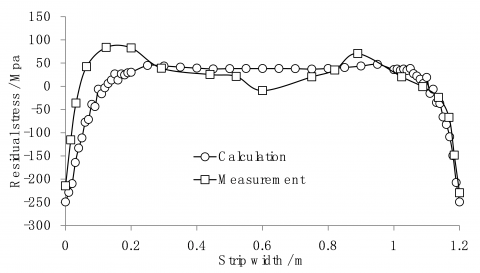Figure 8. Residual stress calculated by FEM and measured by X-ray diffract meter

4. Effect of Two Different Processes on Residual Stress

There are many different processes for residual stress reduction, such as reducing the initial temperature difference, the sparse cooling, the edge masking and rear section cooling. The edge masking is more popular in hot strip production, but the reduction is not obvious. Experience shows that the strip transverse initial temperature difference has a great influence on residual stress. Therefore, residual stress affected by edge masking and transverse initial temperature difference is compared in this section.

4.1 Effect of edge masking on residual stress

Three kinds of edge masking conditions are designed, no masking, 0.2m edge masking and 0.3m edge masking. The initial conditions of the original model are constant, and the boundary conditions are modified. The convection heat transfer coefficient of the strip surface is changed, and the corresponding model of the three conditions is obtained.

Coiling temperatures of three edge masking conditions are shown in Fig.10. It can be seen that temperature drop is smaller when the edge masking is larger. Transverse temperature of strip is abnormal high spot in edge which will be harmful for performance and cause local buckling in cold rolling.

## 9.png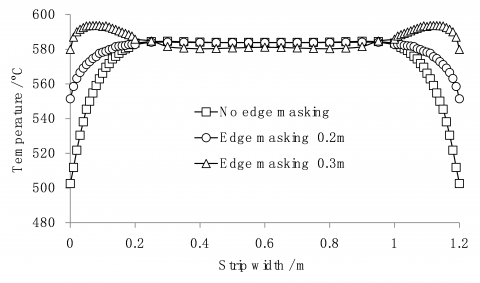Figure 9. Coiling temperatures of three edge masking conditions

Fig.10 shows the stress calculation results under different edge masking conditions. With 0.2m edge masking, the equivalent stress and stress along the strip length are less than the non-masking condition, but with the 0.3m edge masking, equivalent stress is 512.33MPa in the which is more greater than the yield stress and leads to buckling. In strip length direction, strip central stress is positive and tensile stress, otherwise, in strip edge, the stress is negative, and the strip is easy to cause edge wave in 0.3m edge masking according to shape theory. The residual stress of the strip can be reduced by using the edge masking method with proper masking value, otherwise, it will lead to bad strip performance and shape wave before the coiling machine.

## 10.pngFigure 10. Stress calculation results under different edge masking conditions

4.2 Effect of strip transverse initial temperature on residual stress

The initial temperature difference between the edge and the middle part of the strip before laminar cooling will make the difference phase transformation time, which will cause different cooling rate, uniform performance and residual stress. By modifying the initial conditions and boundary conditions, the finite element model of different initial temperature difference is established to study the influence of different temperature on the residual stress in the laminar cooling process. The three kinds of temperature difference between edge and middle strip are 30ºC, 80 ºC and 120 ºC respectively.

Fig. 11 shows the residual stress distribution in the strip after laminar cooling. As can be seen from the diagram, the residual stress in the edge of the strip is larger with the increase of the temperature difference. When the initial temperature difference is 120 ºC, the equivalent stress of the strip is 445.92MPa, which is far greater than the yield stress of the temperature. When the temperature difference is 30 ºC, the equivalent stress of the strip is 106.96MPa, which is far less than the yield stress. The big temperature difference is easy to cause edge wave defect.

## 11.png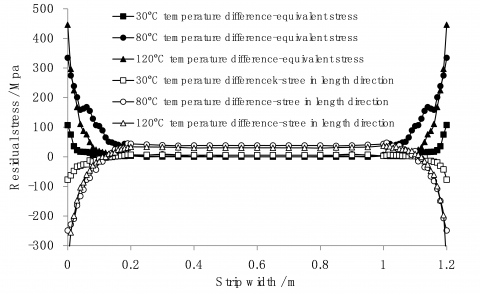Figure 11. Stress calculation results under different initial temperature

4.3 Reduction of residual stress in different conditions

Residual stress reduction in the two processes are compared in Table 1, As can be seen from the table, when the initial edge temperature difference controlled to 30 ºC, the equivalent stress of the strip edge is reduced by 38.17%, when the edge masking is 0.2m, the equivalent stress of the strip edge is reduced by 16.38%. The results show that edge temperature control and edge masking are both effective on residual stress reduction and the initial temperature difference of the strip is more significant.

Table 1. Residual stress reduction in the two processes

 NO. Temperature difference Edge masking Equivalent stress Stress reduction 1 60 ºC - 334.1Mpa - 2 30 ºC - 206.6Mpa 38.17% 3 60 ºC 0.2m 279.4Mpa 16.38%
5. Testing Between Edge Wave and Initial Temperature Difference

In order to verify the effect of strip transverse initial temperature on residual stress, experiment on skin pass mill is carried out. Because the reasons of edge wave are complicate, a lot of strip samples are obtained with less shape defect after finishing mill, only considering the edge wave caused by uniform transverse temperature or cooling rate. In the experiment, the number of strip is 116 and the point in strip is 3077.

Fig. 12 and Fig. 13 show the initial temperature difference and shape defect. According to the comparison of the two graphs, the wave is mainly concentrated in the region of high temperature difference from 50 ºC to 55 ºC, and good shape is mainly concentrated in the region of high temperature difference from 35 ºC to 40 ºC. Therefore, the temperature difference of the edge wave is greater.

## 12.pngFigure 12. Initial temperature difference distribution of shape defect strip samples

## 13.png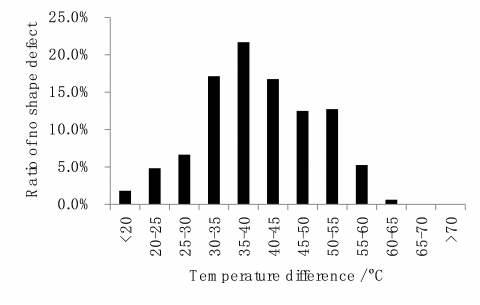Figure 13. Initial temperature difference distribution of good shape defect strip samples

6. Summary

1) Phase transformation is necessary for temperature field calculation in hot strip laminar cooling. The coupled model of temperature, phase transformation and stress can be used for laminar cooling process numerical simulation. At the same time, experimental verification methods are important for the accuracy of the model, including temperature testing, phase transformation structure, and residual stress testing.

2) Edge masking and improving initial temperature difference are also benefit for residual stress reduction, and initial temperature difference is more significant. An example is given to improve the strip residual stress by improving theinitial temperature difference distribution, and the correctness of the proposed method and conclusion is verified.

Acknowledgement

This work was supported in part by National Natural Science Funds of China (51404021), Fundamental Research Funds for the Central Universities (FRF-TP-14-103A2) and Beijing Municipal Natural Science Foundation (3154035).

References

1. G. Yang, Y.U. Ming and G.D. Wang, “Heat transfer of hot strip during ultra-fast cooling,” Journal of Northeastern University, vol.27, pp.406-409, 2006. DOI: 10.3321/j.issn:1005-3026.2006.04.014.

2. G.D. Wang, “The new generation TMCP with the key technology of ultra-fast cooling,” Shanghai Metals, vol.30, pp.1-5, 2008. DOI: 10.3969/j.issn.1001-7208.2008.02.001.

3. Q.D. Zhang, B. Li and X.F. Zhang, “Research on the behavior and effects of flatness control in strip temper rolling process,” Journal of Mechanical Engineering, vol. 50, pp. 45-52, 2014. DOI: 10.3901/JME.2014.08.045.

4. X.D. Wang, A.R. He and Q. Yang, “Temperature and phase transformation coupling prediction model of hot rolled strip cooled on the run-out table,” Journal of University of Science and Technology Beijing, vol. 28, pp. 964-968, 2006. DOI: 10.3321/j.issn:1001-053X.2006.10.013.

5. Y.L. Chen, W. Yu and L. Su, “Analysis of residual stress in hot rolled steel strip during laminar cooling,” Transactions of Materials and Heat Treatment, vol. 31, pp. 155-160, 2010. DOI: 10.13289/j.issn.1009-6264.2010.06.014.

6. H.L. Yi, X. Yang and Z.Y. Liu, “Microstructures and properties of 780 MPa engineering machinery steel under ultra-fast cooling process,” Journal of Northeastern University, vol. 33, pp. 1578-1581, 2012. DOI: 10.3321/j.issn:1005-3026.2009.10.014.

7. H. Yoshida, “Analysis of flatness of hot rolled steel strip after cooling,” Transactions ISIJ, vol. 24, pp. 212-220, 1984. DOI: 10.2355/isijinternational1966.24.212.

8. H.N. Han, S.H. Park, “Model for cooling and phase transformation behavior of transformation induced plasticity steel on run out table in hot strip mill,” Mater. Sci. Technol, vol. 17, pp. 721-726, 2001. DOI: 10.1016/S0924-0136(02)00454-5.

9. H.N. Han, J.K. Lee and H.J. Kim, “Model for deformation, temperature and phase transformation behavior of steels on run-out table in hot strip mill,” J. Mater. Process. Technol., vol. 128, pp. 216-225, 2002. DOI: 10.1016/S0924-0136(02)00454-5.